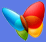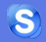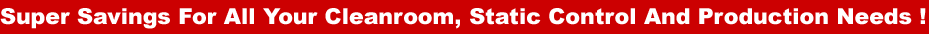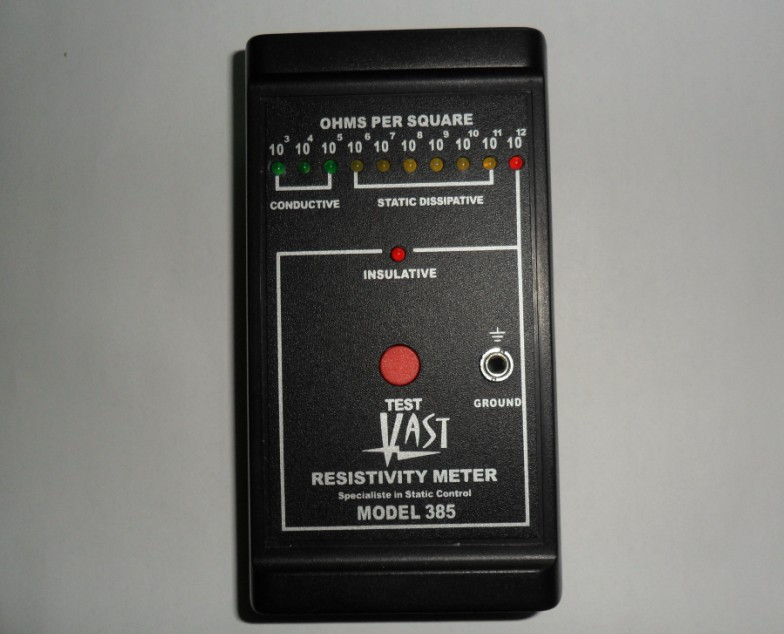ESD Tester

Surface Resistivity Meter
385This compact meter measures both Surface Resistivity and Resistance to Ground with a Test Range From 10^3 ohms per square to 10^12 ohms per square, with accuracy + or – 1/2 Decade.

The 385 gives simple repeatable measurements of Conductive, Static Dissipative and Insulative surfaces by utilizing the ASTM standard D-257 method of parallel bar sensing.

Easy to Operate

To measure surface resistivity, place the meter onto the surface that requires testing, press the red test button and hold. The LED that illuminates constantly is the decade measured.

 10^3 = 1 kilohm Green LED 10^4 = 10 kilohms Green LED 10^5 = 100 kilohms Green LED 10^6 = 1 meg ohm Yellow LED 10^7 = 10 meg ohms Yellow LED 10^8 = 100 meg ohms Yellow LED 10^9 = 1000 meg ohms Yellow LED 10^10 = 10,000 meg ohms Yellow LED 10^11 = 100,000 meg ohms Yellow LED 10^12 = 1,000,000 meg ohms Red LED > 10^12 = Insulative Red LED

Checking Resistance to ground

Insert the ground cord into the ground socket. This isolates the test probe on the right side of the meter (the same side as the socket), connect the alligator clip to your ground connection.

Place the meter onto the surface to be tested, press and hold the test button. The LED that remains illuminated is the decade measured. The measurement will be resistance to ground in ohms instead of ohms per square.

Specification

 Power Supply 9 volt PP3 alkaline battery Test Voltage Nominal 9 volts Relativumidity 0% to 90% (non-condensing) Repeatability +/-5% Accuracy +/-10% Weight 170g Size 130 x 70 x 25mm

Copyright 1999 – 2010 Shenzhen Everclean Technology    粤ICP备10100118号-1
Site Design by www.ni8.net.cn